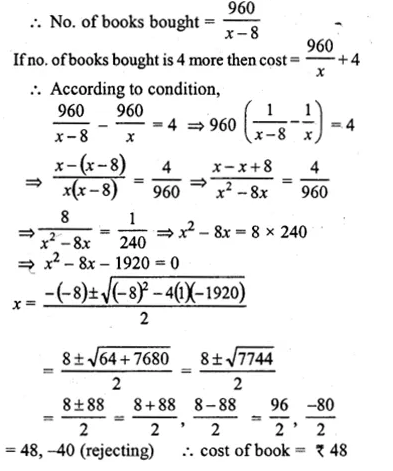Guru

# A shopkeeper buys a certain number of books for Rs 960. If the cost per book was Rs 8 less, the number of books that could be bought for Rs 960 would be 4 more. Taking the original cost of each book to be Rs x, write an equation in x and solve it to find the original cost of each book.

• 0

This question has been taken from Book:- ML aggarwal, Avichal publication, class10th, quadratic equation in one variable, chapter 5, exercise 5.5
This is an important ques in 2013 exam.

A shopkeeper buys a certain number of books for Rs 960. If the cost per book was Rs 8 less, the number of books that could be bought for Rs 960 would be 4 more. Taking the original cost of each book to be Rs x, write an equation in x and solve it to find the original cost of each book.
Question no37. , ML Aggarwal, chapter 5, exercise 5.5, quadratic equation in one variable, ICSE board,

Share